# 2-way sms sends undesireable results with php

 2-way sms sends undesireable results with php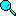Search

 SMS & MMS Technical Forum » NowSMS Support - SMS Issues (Product Support Only) » Archive through May 07, 2008 » 2-way sms sends undesireable results with php « Previous || Next »
Author Message
ebiti edide
New member

Post Number: 1
Registered: 01-2008
 Posted on Monday, January 14, 2008 - 08:59 am:

These 2-way sms code written with php works well when tested on a webserver with html form but sends undesireable results when tested with now sms and a gsm modem.

i'm attaching herewith everthing you will need to test run this code yourself so u can figure out the prohlem.

This code is for a 2-way quiz game.

-A player enters the game by sending sms "warzone"

-the code sends him a question immediately

-the players returns the answers. if the answer is correct, the code sends the player another question.

-this process is repeated untill the player either fails or reaches level 10 of the game where he or she wins a price.

The php code:

<?php

//connect to database and select diamondquiz_db
include('connect_diamondquiz.php');

function check_for_player_step(\$phoneno){
\$sql="select step from step_table
where phoneno = '\$phoneno'";
\$result = @mysql_query(\$sql);
if (!\$result) {
die('<p>Error performing query: ' . mysql_error() . ' </p>');
}
\$row = mysql_fetch_array(\$result);
\$player_step = \$row['step'];
return \$player_step;
}
function delete_player_from_step_table(\$phoneno){
\$sql="delete from step_table where phoneno = '\$phoneno'";
\$result = @mysql_query(\$sql);
if (!\$result) {
die('<p>Error unable to delete from step_table: ' . mysql_error() . ' </p>');
}
}
function delete_player_from_time_table(\$phoneno){
\$sql="delete from time_table where phoneno = '\$phoneno'";
\$result = @mysql_query(\$sql);
if (!\$result) {
die('<p>Error unable to delete from time_table: ' . mysql_error() . ' </p>');
}
}
function check_elasped_time(\$phoneno){
\$sql="select time_to_sec(timesent) as timesent from time_table
where phoneno = '\$phoneno'";
\$result = @mysql_query(\$sql);
if (!\$result) {
die('<p>Error performing query: ' . mysql_error() . ' </p>');
}
\$row = mysql_fetch_array(\$result);
\$time_sent = \$row['timesent'];

\$sql1="select time_to_sec(curtime()) as recvtime";
\$result1 = @mysql_query(\$sql1);
if (!\$result1) {
die('<p>Error performing query: ' . mysql_error() . ' </p>');
}
\$row1 = mysql_fetch_array(\$result1);
\$recievedtime = \$row1['recvtime'];

\$time_in_mins = ((\$recievedtime/60)-(\$time_sent/60));
return \$time_in_mins;
}
\$sql="select quizno from ongoing_table where phoneno = '\$phoneno'";
\$result = @mysql_query(\$sql);
if (!\$result) {
die('<p>Error performing query: ' . mysql_error() . ' </p>');
}
\$row = mysql_fetch_array(\$result);
\$quiz_no = \$row['quizno'];

\$sql1="select answer from quiz_table where quizno = '\$quiz_no'";
\$result1 = @mysql_query(\$sql1);
if (!\$result1) {
die('<p>Error performing query: ' . mysql_error() . ' </p>');
}
\$row1 = mysql_fetch_array(\$result1);
}
function record_step_in_step_table(\$phoneno,\$step){
\$sql="insert into step_table values('\$phoneno','\$step')";
\$result = @mysql_query(\$sql);
if (!\$result) {
die('<p>Error inserting data into step_table: ' . mysql_error() . ' </p>');
}
}
function record_step_two_for_player(\$phoneno){
\$sql="insert into step_table values('\$phoneno','2')";
\$result = @mysql_query(\$sql);
if (!\$result) {
die('<p>Error inserting step two for player: ' . mysql_error() . ' </p>');
}
}
function record_players_phoneno_and_quizno_in_ongoing_table(\$phoneno,\$quizno){
\$sql="insert into ongoing_table values('\$phoneno','\$quizno')";
\$result = @mysql_query(\$sql);
if (!\$result) {
die('<p>Error inserting data into ongoing_table: ' . mysql_error() . ' </p>');
}
}
function record_players_phoneno_and_timesent_in_time_table(\$phoneno){
\$sql="insert into time_table values('\$phoneno',curtime())";
\$result = @mysql_query(\$sql);
if (!\$result) {
die('<p>Error inserting data into time_table: ' . mysql_error() . ' </p>');
}
}
\$result = @mysql_query(\$sql);
if (!\$result) {
die('<p>Error inserting data into check_if_quizno_already_exist_table: ' . mysql_error() . ' </p>');
}
}
function delete_player_from_ongoing_table(\$phoneno){
\$sql="delete from ongoing_table where phoneno = '\$phoneno'";
\$result = @mysql_query(\$sql);
if (!\$result) {
die('<p>Error unable to delete from ongoing_table: ' . mysql_error() . ' </p>');
}
}
function randomly_select_quizno(\$phoneno){
\$sql="select quizno from quiz_table";
\$result = @mysql_query(\$sql);
if (!\$result) {
die('<p>Error performing query: ' . mysql_error() . ' </p>');
}
\$count_of_quiz_rows = @mysql_num_rows(\$result);

//initialize count for outer loop
\$count = 0;
//use 100 as a seed value
while (\$count < 100){
//select a random number b/w 1 and the
//total count of quiz in quiz table
\$random =rand(1,\$count_of_quiz_rows);
\$sql1="select quizno from check_if_quizno_already_exist_table where phoneno = '\$phoneno'";
\$result1 = @mysql_query(\$sql1);
if (!\$result1) {
die('<p>Error performing query: ' . mysql_error() . ' </p>');
}
//find total count of quiz in quiz table
\$count_rows = @mysql_num_rows(\$result1);
//initialize count for inner loop
\$count2 = 0;
//while count is less than total count of quiz
while (\$count2 < \$count_rows){
//collect the already existing quizno array
//into variable row one at a time
\$row = mysql_fetch_array(\$result1);
//compare the quizno to the randam
//no generated
\$res = strcmp(\$row['quizno'],\$random);
//if same
if (\$res == 0){
//break out of the second loop
break;
}
//increment counter for second loop
\$count2++;
}
//if inner loop checked through the resultset
//and couldn't find any value equal the random
//number,then return that randam number value.
if (\$count2 == \$count_rows and \$res != 0){
return \$random;
exit();
}
//increment counter for second loop
\$count++;
}
}
function randomly_select_quizno_for_war_zone(\$phoneno){
\$sql="select quizno from quiz_table";
\$result = @mysql_query(\$sql);
if (!\$result) {
die('<p>Error performing query: ' . mysql_error() . ' </p>');
}
\$count_of_quiz_rows = @mysql_num_rows(\$result);
\$random =rand(1,\$count_of_quiz_rows);
return \$random;
}
function insert_players_record_into_winners_table(\$phoneno,\$playerstep){
\$sql="insert into winners_table values('\$phoneno','\$playerstep',curdate())";
\$result = @mysql_query(\$sql);
if (!\$result) {
die('<p>Error inserting data into winners_table: ' . mysql_error() . ' </p>');
}
}
function select_quizno_from_quiz_table(\$phoneno){
\$sql="select quizno from ongoing_table where phoneno = '\$phoneno'";
\$result = @mysql_query(\$sql);
if (!\$result) {
die('<p>Error performing query: ' . mysql_error() . ' </p>');
}
\$row = mysql_fetch_array(\$result);
\$quiz_no = \$row['quizno'];
return \$quiz_no;
}
function players_step_less_than_or_equal_to_four(\$sender,\$message,\$playerstep){
//step is assigned database step
\$count = \$playerstep;
if (\$count == 5){
echo "u are in step 5. u can decide to walk away and claim your prize. if u play and fail, u go back to war zone level 2. To continue, in less than 2 mins text upper level\r\n";
exit();
}
\$time_elasped = check_elasped_time(\$sender);
/*if player's time has expired,send a response
if (\$time_elasped >= 3) {
echo "your time expired. Game Over!!!\r\n";
//select quizno from quiz table
\$quizno = select_quizno_from_quiz_table(\$sender);
and the reason the player stop to play in th players table*/
\$sql="insert into players_table values('\$sender','\$playerstep','\$quizno','\$message',curdate(),curtime(),'your time expired')";
\$result = @mysql_query(\$sql);
if (!\$result) {
die('<p>Error inserting data into players_table: ' . mysql_error() . ' </p>');
}
//delete player from the step table
delete_player_from_step_table(\$sender);
//delete player from the time table
delete_player_from_time_table(\$sender);
//delete player from ongoing table
delete_player_from_ongoing_table(\$sender);
//exit
exit();
}
/*use the quiz no in the on-goning table to check
/*if player's answer is wrong,send a response
if (\$res < 0 or \$res > 0){
//select quizno from quiz table
\$quizno = select_quizno_from_quiz_table(\$sender);
and the reason the player stop to play in th players table*/
\$sql="insert into players_table values('\$sender','\$playerstep','\$quizno','\$message',curdate(),curtime(),'incorre ct answer')";
\$result = @mysql_query(\$sql);
if (!\$result) {
die('<p>Error inserting data into players_table: ' . mysql_error() . ' </p>');
}
//delete player from the step table
delete_player_from_step_table(\$sender);
//delete player from the time table
delete_player_from_time_table(\$sender);
//delete player from ongoing table
delete_player_from_ongoing_table(\$sender);
//exit
exit();
}
//delete player from the step table
delete_player_from_step_table(\$sender);
//delete player from the time table
delete_player_from_time_table(\$sender);
//delete quiz no,phoneno in on-going table
delete_player_from_ongoing_table(\$sender);
//record step in the steps table
\$step = \$count + 1;
record_step_in_step_table(\$sender,\$step);
//randomly select quiz from the quiz table
\$quizno = randomly_select_quizno(\$sender);
\$sql="select quiz from quiz_table where quizno = '\$quizno'";
\$result = @mysql_query(\$sql);
if (!\$result) {
die('<p>Error performing query: ' . mysql_error() . ' </p>');
}
\$row = mysql_fetch_array(\$result);
\$quiz = \$row['quiz'];
//send quiz to the player
echo \$quiz;
//record quizno in check if quiz no already exist table
//record quiz no and phoneno in the on-going table
record_players_phoneno_and_quizno_in_ongoing_table(\$sender,\$quizno);
//record phoneno and timesent in the time table
record_players_phoneno_and_timesent_in_time_table(\$sender);
}
function upper_level(\$sender,\$message,\$playerstep){
//step is assigned database step
\$count = \$playerstep;
if (\$count == 7){
echo "u are in step 7 and u are about to enter step 8. u can walk away now cos if u play and fail, u go back to level 2. To continue, in less than 1 min text medal of honor\r\n";
exit();
}
//check time elasped b/w sending of question and receiving of answer
\$time_elasped = check_elasped_time(\$sender);
/*if the time expired, send a response
if (\$time_elasped >= 2) {
echo "your time expired. Game Over!!!\r\n";
//select quizno from quiz table
\$quizno = select_quizno_from_quiz_table(\$sender);
/*record the player's phoneno,answer,date,time and they
reason the player stopped to play in the players table*/
\$sql="insert into players_table values('\$sender','\$playerstep','\$quizno','\$message',curdate(),curtime(),'your time expired')";
\$result = @mysql_query(\$sql);
if (!\$result) {
die('<p>Error inserting data into players_table: ' . mysql_error() . ' </p>');
}
//record the player's phoneno,step,date in the winner's table
insert_players_record_into_winners_table(\$sender,\$playerstep);
//delete player from the step table
delete_player_from_step_table(\$sender);
//delete player from the time table
delete_player_from_time_table(\$sender);
//delete quiz no,phoneno in on-going table
delete_player_from_ongoing_table(\$sender);
//exit
exit();
}
/*use the quiz no in the on-goning table to check
/*if player's answer is wrong,send a response
"incorrect answer, you are back at level 2.We are sending a quiz shortly"*/
if (\$res > 0 or \$res < 0){
echo "you are back at level 2.We are sending a quiz shortly\r\n";
//delete player from the step table
delete_player_from_step_table(\$sender);
//delete player from the time table
delete_player_from_time_table(\$sender);
//record step 2 for player in the steps table
record_step_two_for_player(\$sender);
//delete quiz no,phoneno in on-going table
delete_player_from_ongoing_table(\$sender);
//randomly select quiz from the quiz table
\$quizno = randomly_select_quizno(\$sender);
\$sql="select quiz from quiz_table where quizno = '\$quizno'";
\$result = @mysql_query(\$sql);
if (!\$result) {
die('<p>Error performing query: ' . mysql_error() . ' </p>');
}
\$row = mysql_fetch_array(\$result);
\$quiz = \$row['quiz'];
//send quiz to the player
echo \$quiz;
//record quizno in check if quiz no already exist table
//record quiz no and phoneno in the on-going table
record_players_phoneno_and_quizno_in_ongoing_table(\$sender,\$quizno);
//record phoneno and timesent in the time table
record_players_phoneno_and_timesent_in_time_table(\$sender);
//exit
exit();
}
/*if player's time didn't expire and

//delete player from the step table
delete_player_from_step_table(\$sender);
//record step in the steps table
\$step = \$count + 1;
record_step_in_step_table(\$sender,\$step);
//delete player from the time table
delete_player_from_time_table(\$sender);
//delete quiz no,phoneno in on-going table
delete_player_from_ongoing_table(\$sender);
//randomly select quiz from the quiz table
\$quizno = randomly_select_quizno(\$sender);
\$sql="select quiz from quiz_table where quizno = '\$quizno'";
\$result = @mysql_query(\$sql);
if (!\$result) {
die('<p>Error performing query: ' . mysql_error() . ' </p>');
}
\$row = mysql_fetch_array(\$result);
\$quiz = \$row['quiz'];
echo \$quiz;
//record quizno in check if quiz no already exist table
//record quiz no and phoneno in the on-going table
record_players_phoneno_and_quizno_in_ongoing_table(\$sender,\$quizno);
//record phoneno and timesent in the time table
record_players_phoneno_and_timesent_in_time_table(\$sender);
}
function medal_of_honor(\$sender,\$message,\$playerstep){
//step is assigned database step
\$count = \$playerstep;
if (\$count == 10){
echo "Congrats, u are at level 10. U won.\r\n";
exit();
}
//check time elasped b/w sending of question and receiving of answer
\$time_elasped = check_elasped_time(\$sender);
/*if the time expired, send a response
if (\$time_elasped >= 1) {
echo "your time expired. Game Over!!!\r\n";
//select quizno from quiz table
\$quizno = select_quizno_from_quiz_table(\$sender);
/*record the player's phoneno,answer,date,time and they
reason the player stopped to play in the players table*/
\$sql="insert into players_table values('\$sender','\$playerstep','\$quizno','\$message',curdate(),curtime(),'your time expired')";
\$result = @mysql_query(\$sql);
if (!\$result) {
die('<p>Error inserting data into players_table: ' . mysql_error() . ' </p>');
}
//record the player's phoneno,step,date in the winner's table
insert_players_record_into_winners_table(\$sender,\$playerstep);
//delete player from the step table
delete_player_from_step_table(\$sender);
//delete player from the time table
delete_player_from_time_table(\$sender);
//delete quiz no,phoneno in on-going table
delete_player_from_ongoing_table(\$sender);
//exit
exit();
}
/*use the quiz no in the on-goning table to check
/*if player's answer is wrong,send a response
"incorrect answer, you are back at level 2.We are sending a quiz shortly"*/
if (\$res > 0 or \$res < 0){
echo "you are back at level 2.We are sending a quiz shortly\r\n";
//delete player from the step table
delete_player_from_step_table(\$sender);
//delete player from the time table
delete_player_from_time_table(\$sender);
//record step 2 for player in the steps table
record_step_two_for_player(\$sender);
//delete quiz no,phoneno in on-going table
delete_player_from_ongoing_table(\$sender);
//randomly select quiz from the quiz table
\$quizno = randomly_select_quizno(\$sender);
\$sql="select quiz from quiz_table where quizno = '\$quizno'";
\$result = @mysql_query(\$sql);
if (!\$result) {
die('<p>Error performing query: ' . mysql_error() . ' </p>');
}
\$row = mysql_fetch_array(\$result);
\$quiz = \$row['quiz'];
//send quiz to the player
echo \$quiz;
//record quizno in check if quiz no already exist table
//record quiz no and phoneno in the on-going table
record_players_phoneno_and_quizno_in_ongoing_table(\$sender,\$quizno);
//record phoneno and timesent in the time table
record_players_phoneno_and_timesent_in_time_table(\$sender);
//exit
exit();
}
/*if player's time didn't expire and

//delete player from the step table
delete_player_from_step_table(\$sender);
//record step in the steps table
\$step = \$count + 1;
record_step_in_step_table(\$sender,\$step);
//delete player from the time table
delete_player_from_time_table(\$sender);
//delete quiz no,phoneno in on-going table
delete_player_from_ongoing_table(\$sender);
//randomly select quiz from the quiz table
\$quizno = randomly_select_quizno(\$sender);
\$sql="select quiz from quiz_table where quizno = '\$quizno'";
\$result = @mysql_query(\$sql);
if (!\$result) {
die('<p>Error performing query: ' . mysql_error() . ' </p>');
}
\$row = mysql_fetch_array(\$result);
\$quiz = \$row['quiz'];
echo \$quiz;
//record quizno in check if quiz no already exist table
//record quiz no and phoneno in the on-going table
record_players_phoneno_and_quizno_in_ongoing_table(\$sender,\$quizno);
//record phoneno and timesent in the time table
record_players_phoneno_and_timesent_in_time_table(\$sender);
}
function just_started(\$sender){
//initialize step to zero
\$step = 1;
//record phoneno,step in steps table
record_step_in_step_table(\$sender,\$step);
//randomly select quiz from quiz table
\$quizno = randomly_select_quizno_for_war_zone(\$sender);
\$sql="select quiz from quiz_table where quizno = '\$quizno'";
\$result = @mysql_query(\$sql);
if (!\$result) {
die('<p>Error performing query: ' . mysql_error() . ' </p>');
}
\$row = mysql_fetch_array(\$result);
\$quiz = \$row['quiz'];
//send quiz to the player
echo \$quiz;
//record quizno in check if quiz no already exist table
//record quiz no, phoneno in on-going table.
record_players_phoneno_and_quizno_in_ongoing_table(\$sender,\$quizno);
//record phoneno and timesent in the time table
record_players_phoneno_and_timesent_in_time_table(\$sender);
}

\$sender = \$_REQUEST['sender'];
//\$message = \$_REQUEST['msg'];
//\$recievedtime = \$_REQUEST['recvtime'];
/*use the player's phoneno to check for
the player's step in the steps table*/
\$playerstep = check_for_player_step(\$sender);

//if player just started
if (isset(\$_REQUEST['msg'])) {
\$message = \$_REQUEST['msg'];
if (\$message == 'warzone'){
just_started(\$sender);
exit();
}
//if player sent sms upper level and player is in step 5
elseif (\$message == 'upperlevel'){
if (\$playerstep = 5){
//delete player from the step table
delete_player_from_step_table(\$sender);
//initialize step to six
\$step = 6;
//record phoneno,step in steps table
record_step_in_step_table(\$sender,\$step);
//delete player from the time table
delete_player_from_time_table(\$sender);
//delete quiz no,phoneno in on-going table
delete_player_from_ongoing_table(\$sender);
//randomly select quiz from quiz table
\$quizno = randomly_select_quizno(\$sender);
\$sql="select quiz from quiz_table where quizno = '\$quizno'";
\$result = @mysql_query(\$sql);
if (!\$result) {
die('<p>Error performing query: ' . mysql_error() . ' </p>');
}
\$row = mysql_fetch_array(\$result);
\$quiz = \$row['quiz'];
//send quiz to the player
echo \$quiz;
//record quizno in check if quiz no already exist table
//record quiz no, phoneno in on-going table.
record_players_phoneno_and_quizno_in_ongoing_table(\$sender,\$quizno);
//record phoneno and timesent in the time table
record_players_phoneno_and_timesent_in_time_table(\$sender);
exit();
}
}
//if player sent sms medal of honor and player is in step 6
elseif (\$message == 'medalofhonor'){
if (\$playerstep = 7){
//delete player from the step table
delete_player_from_step_table(\$sender);
//initialize step to zero
\$step = 8;
//record phoneno,step in steps table
record_step_in_step_table(\$sender,\$step);
//delete player from the time table
delete_player_from_time_table(\$sender);
//delete quiz no,phoneno in on-going table
delete_player_from_ongoing_table(\$sender);
//randomly select quiz from quiz table
\$quizno = randomly_select_quizno(\$sender);
\$sql="select quiz from quiz_table where quizno = '\$quizno'";
\$result = @mysql_query(\$sql);
if (!\$result) {
die('<p>Error performing query: ' . mysql_error() . ' </p>');
}
\$row = mysql_fetch_array(\$result);
\$quiz = \$row['quiz'];
//send quiz to the player
echo \$quiz;
//record quizno in check if quiz no already exist table
//record quiz no, phoneno in on-going table.
record_players_phoneno_and_quizno_in_ongoing_table(\$sender,\$quizno);
//record phoneno and timesent in the time table
record_players_phoneno_and_timesent_in_time_table(\$sender);
exit();
}
}
//if player is still in upper level and between step 4 to 6
elseif (\$playerstep >= 6 and \$playerstep <= 7){
upper_level(\$sender,\$message,\$playerstep);
exit();
}
/*if the players's step is greater than or equal to 7 and less than 10,call
medal of honor function else send response u are not in medal of honor.*/
elseif (\$playerstep >= 8 and \$playerstep < 11){
medal_of_honor(\$sender,\$message,\$playerstep);
}
/*if the players's step is less than or equal to five,check
wether player's time elaspsed is 3 mins in the time's table*/
else{
players_step_less_than_or_equal_to_four(\$sender,\$message,\$playerstep);
exit();
}
}else{
echo "don't send an empty text";
}
?>

The mysql tables with all the data you will need to test run the code:

drop table if exists step_table;
create table step_table
(phoneno varchar(15) not null,
step int not null,
primary key(phoneno));

drop table if exists time_table;
create table time_table
(phoneno varchar(15) not null,
timesent time not null,
primary key(phoneno));

drop table if exists ongoing_table;
create table ongoing_table
(phoneno varchar(15) not null,
quizno int(5) not null,
primary key(phoneno));

drop table if exists awaiting_ans;
create table awaiting_ans
(session_id varchar(35) not null,
staff varchar(50) not null);

drop table if exists winners_table;
create table winners_table
(phoneno varchar(15) not null,
step int not null,
playing_date date not null,
primary key(phoneno));

drop table if exists players_table;
create table players_table
(phoneno varchar(15) not null,
step int not null,
quizno int(5) not null,
curdate date not null,
curtime time not null,
reson_player_stopped varchar(20) not null,
primary key(phoneno));

(phoneno varchar(15) not null,
quizno int(5) not null);

drop table if exists quiz_table;
create table quiz_table
(quizno int(5) not null auto_increment,
quiz text not null,
primary key(quizno),
unique id(quizno));

insert into quiz_table values ('1','who was Nigeria first women speaker (a)mageret ekpo (b)Mrs Ette (c)Stella Obasanjo','B');
insert into quiz_table values ('2','How was dele giwa killed (a)hanging (b)car accident (c)bomb blast','C');
insert into quiz_table values ('3','what is the capital of crs (a)obudu (b)ikom (c)calabar','C');
insert into quiz_table values ('4','At what age did bob marley die (a)1957 (b)1947 (c)1967','C');
insert into quiz_table values ('5','which is the network with the largest coverage in Nig. (a)mtn (b)glo (c)ceiltel','B');
insert into quiz_table values ('6','who was the first president of Nigeria (a)Aguyi Ironsi (b)Azikiwe (c)Tarfa Balewa','B');
insert into quiz_table values ('7','Which election was acclaimed the friest Nigeria election (a)2007 (b)1992 (c)1999','B');
insert into quiz_table values ('8','Which year is Nigerian government set to drop the value of Naira (a)2020 (b)2010 (c)2008','C');
insert into quiz_table values ('9','who was the captain of the first Nigerian soccer team to win the U17 world cup (a)Odegbami (b)Ike shoromu (c)Siasia','A');
insert into quiz_table values ('10','How many years rememberance service of princess Diana death held in 2007 (a)12th (b)10th (C)8th','B');
insert into quiz_table values ('11','How many matches did Nigeria loose at the 1994 world cup (a)1 (b)2 (C)3','B');
insert into quiz_table values ('12','Which year did bill gates retire actively from Micorsoft (a)2005 (b)2007 (c)2006','B');

The html form to find out if the code is working well or not:

<!DOCTYPE html PUBLIC "-//W3C//DTD XHTML 1.0 Transitional//EN" "http://www.w3.org/TR/xhtml1/DTD/xhtml1-transitional.dtd">
<html xmlns="http://www.w3.org/1999/xhtml">
<meta http-equiv="Content-Type" content="text/html; charset=iso-8859-1" />
<title>Untitled Document</title>

<body>
<form id="form1" name="form1" method="post" action="diamondquiz.php">
<label>
<input type="text" name="sender" />
</label>
sender
<p>
<label>
</label>
<p>
<label>
<input type="text" name="msg" />
</label>
message</p>
<p>
<label></label>
<label>
<input type="submit" name="Submit" value="Submit" />
</label>
</p>
</form>
</body>
</html>

Please note that i'm using the 60 days trial version of your software with the hope to get a license if it's okay for me. Meanwhile the owners of these project are already on my neck to deliver, so i will be extremely greatlyful for your help.

my email once more is e.edide@yahoo.com in case u didn't get it right when i registered.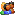Login / Register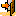Logout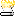Last 30 Days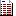Topics